2021 NBA Sixth Man of the Year Odds
+600
6 to 1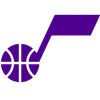Jordan Clarkson
8.0% implied probability

+1000
10 to 1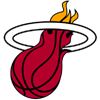Tyler Herro
5.1% implied probability

+1300
13 to 1Joe Ingles
4.0% implied probability

+1500
15 to 1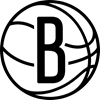Patty Mills
3.5% implied probability

+1600
16 to 1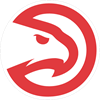Kevin Huerter
3.3% implied probability

+1700
17 to 1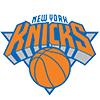Derrick Rose
3.1% implied probability

+1800
18 to 1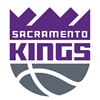Tyrese Haliburton
2.9% implied probability

+2000
20 to 1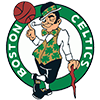Dennis Schroder
2.7% implied probability

No changes have been recorded yet.
+2000
20 to 1Jordan Poole
2.7% implied probability

+2200
22 to 1Jalen Brunson
2.4% implied probability

+2200
22 to 1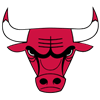Coby White
2.4% implied probability

+2200
22 to 1Goran Dragic
2.4% implied probability

+2500
25 to 1Cameron Payne
2.1% implied probability

No changes have been recorded yet.
+2500
25 to 1Terrence Ross
2.1% implied probability

+2500
25 to 1Malik Beasley
2.1% implied probability

No changes have been recorded yet.
+3500
35 to 1T.J. McConnell
1.5% implied probability

+3500
35 to 1Bobby Portis
1.5% implied probability

+3500
35 to 1Alex Caruso
1.5% implied probability

+3500
35 to 1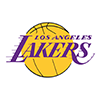Kendrick Nunn
1.5% implied probability

+3500
35 to 1Buddy Hield
1.5% implied probability

+3500
35 to 1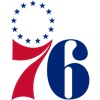Shake Milton
1.5% implied probability

+3500
35 to 1Malik Monk
1.5% implied probability

No changes have been recorded yet.
+3500
35 to 1Terance Mann
1.5% implied probability

+4000
40 to 1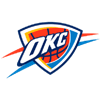Tre Mann
1.4% implied probability

No changes have been recorded yet.
+4000
40 to 1Matisse Thybulle
1.4% implied probability

+4000
40 to 1Chris Boucher
1.4% implied probability

+4500
45 to 1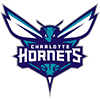Miles Bridges
1.2% implied probability

+4500
45 to 1Cameron Johnson
1.2% implied probability

+5000
50 to 1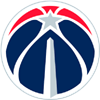Montrezl Harrell
1.1% implied probability

No changes have been recorded yet.
+5000
50 to 1Carmelo Anthony
1.1% implied probability

+5500
55 to 1Lou Williams
1.0% implied probability

+6000
60 to 1Davis Bertans
0.9% implied probability

+6000
60 to 1Tyrese Maxey
0.9% implied probability

No changes have been recorded yet.
+6000
60 to 1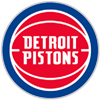Kelly Olynyk
0.9% implied probability

No changes have been recorded yet.
+6500
65 to 1Marvin Bagley
0.8% implied probability

+6500
65 to 1Rodney Hood
0.8% implied probability

+6500
65 to 1Payton Pritchard
0.8% implied probability

+6500
65 to 1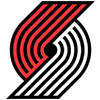Anfernee Simons
0.8% implied probability

+6500
65 to 1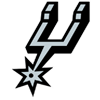Lonnie Walker
0.8% implied probability

+7000
70 to 1Doug McDermott
0.8% implied probability

+7000
70 to 1Luke Kennard
0.8% implied probability

+7000
70 to 1Mitchell Robinson
0.8% implied probability

+7000
70 to 1Danilo Gallinari
0.8% implied probability

+7000
70 to 1Thaddeus Young
0.8% implied probability

+7500
75 to 1De'Anthony Melton
0.7% implied probability

No changes have been recorded yet.
+7500
75 to 1Jeff Green
0.7% implied probability

+7500
75 to 1Juan Toscano-Anderson
0.7% implied probability

+8000
80 to 1Brandon Clarke
0.7% implied probability

+8000
80 to 1Otto Porter
0.7% implied probability

+8000
80 to 1Grayson Allen
0.7% implied probability

+8000
80 to 1Immanuel Quickley
0.7% implied probability

No changes have been recorded yet.
+8000
80 to 1Daniel Gafford
0.7% implied probability

+8000
80 to 1Josh Hart
0.7% implied probability

+9000
90 to 1Eric Bledsoe
0.6% implied probability

+9000
90 to 1Facundo Campazzo
0.6% implied probability

No changes have been recorded yet.
+9000
90 to 1Talen Horton-Tucker
0.6% implied probability

No changes have been recorded yet.
+9000
90 to 1Tomas Satoransky
0.6% implied probability

+9000
90 to 1Bruce Brown
0.6% implied probability

+9000
90 to 1Andre Drummond
0.6% implied probability

+10000
100 to 1Aaron Holiday
0.6% implied probability

+10000
100 to 1Serge Ibaka
0.6% implied probability

+10000
100 to 1Justise Winslow
0.6% implied probability

+10000
100 to 1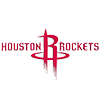Kenyon Martin
0.6% implied probability

+10000
100 to 1Josh Okogie
0.6% implied probability

+10000
100 to 1DeMarcus Cousins
0.6% implied probability

+10000
100 to 1Harrison Barnes
0.6% implied probability

+10000
100 to 1Terence Davis
0.6% implied probability

+13000
130 to 1Jaxson Hayes
0.4% implied probability

+13000
130 to 1Deni Avdija
0.4% implied probability

+13000
130 to 1Sekou Doumbouya
0.4% implied probability

+13000
130 to 1Enes Kanter
0.4% implied probability

+13000
130 to 1Dwight Howard
0.4% implied probability

+13000
130 to 1Hamidou Diallo
0.4% implied probability

+13000
130 to 1Pat Connaughton
0.4% implied probability

+13000
130 to 1Furkan Korkmaz
0.4% implied probability

+13000
130 to 1Jarrett Culver
0.4% implied probability